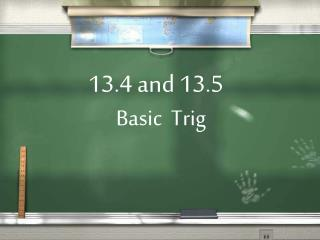# 13.4 and 13.5 - PowerPoint PPT PresentationDownload Presentation13.4 and 13.5

13.4 and 13.5
Download Presentation## 13.4 and 13.5

- - - - - - - - - - - - - - - - - - - - - - - - - - - E N D - - - - - - - - - - - - - - - - - - - - - - - - - - -
##### Presentation Transcript

1. 13.4 and 13.5 Basic Trig

2. Today we will… Find the sine, cosine, and tangent values for angles. We will also use the sine, cosine and tangent to find angles.

3. New Words Trigonometry: the study of the properties of triangles. Trigonometric ratio: ratio of the measures of 2 sides of a triangle.

4. New Words Sine: written sin, read [sahyn] Cosine: written cos, read [koh-sahyn] Tangent: written tan, read [tan- juhnt)

5. New sayings Some old horse, caught another horse, taking oats away. Signs of happiness come after having tankards of ale. Some of her children are having trouble over algebra. Some out houses can actually have totally odorless aromas. SOH CAH TOA (read soh-kuh-toh-uh)

6. Has 3 sides: Hypotenuse Adjacent Opposite Right Triangle The Hypotenuse is always across from the right angle. It is the longest. The other two will depend on what angle we are dealing with

7. Right Triangle The opposite side is across the triangle from the angle. The adjacent side is next to the angle. It is one of the sides of the angle.

10. Sine Sine is the ratio of the opposite side divided by the hypotenuse. C opp hyp 3 5 sin B = 5 3 opp hyp 4 5 sin C = B 4

11. Cosine Cosine is the ratio of the adjacent side divided by the hypotenuse. C adj hyp 4 5 cos B = 5 3 adj hyp 3 5 cos C = B 4

12. Tangent tangent is the ratio of the opposite side divided by the adjacent. C opp adj 3 4 tan B = 5 3 opp adj 4 3 tan C = B 4

13. What’s up with the sayings Some old horse, caught another horse, taking oats away. opp hyp adj hyp opp adj sin = cos = tan =

14. Which ever one you like... Some old horse, caught another horse, taking oats away. Signs of happiness come after having tankards of ale. Some of her children are having trouble over algebra. Some out houses can actually have totally odorless aromas. SOH CAH TOA (read soh-kuh-toh-uh) soak-a-toe-a

15. New Words Angle of depression Angle of elevation

16. Find tan j l 6 j opp adj 3 6 tan J = 3 45 k

17. Find cos j l 6 j adj hyp 6 45 cos J = 3 45 now simplify k

18. Find tan k l 12 j opp adj 12 5 tan K = 5 13 k

19. Find sin k l 12 j opp hyp 12 13 sin K = 5 13 k

20. Find cos k l 12 j adj hyp 5 13 cos K = 5 13 k

21. What ratio can we use? To find a side... What sides do we know? 30 opp adj ? 13 13 tan 30 = ? 13 .5774 = ? .5774 X 13 = ?

22. What ratio can we use? To find a side... What sides do we know? 18 210 adj hyp ? 210 ? cos 18 = ? 210 .9511 = .9511 X 210 = ?

23. What ratio can we use? To find an angle... What sides do we know? 95 opp adj 95 40 tan ? = = 2.375 ? tan-1 2.375 = 67.17 40

24. What ratio can we use? To find an angle... ? What sides do we know? 3078 opp hyp 600 3078 sin ? = = .1949 sin-1 .1949 = 11.24 600

25. Make sure your calculator is in degrees not radians When calculating trig functions, we usually round to 4 decimal places